Lesson 6: Increasing the fifth column

As mentioned in the previous chapter, let's talk about rising that fifth column.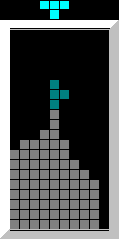The T can only be moved to both sides if the 5th column is the highest.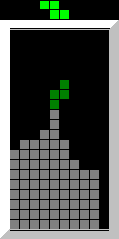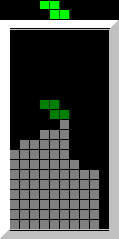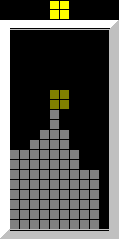The Z and O can be moved to both sides if the 5th or 6th column is the highest. Be careful about using IRS if it's the 6th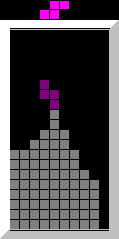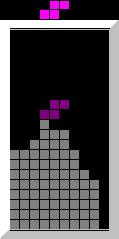The S can be moved to both sides if the 4th or 5th column is the highest. Be sure to use IRS if it's the 4th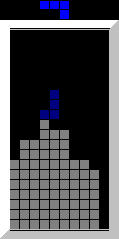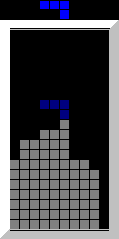The J and L can be moved to both sides if the 4th, 5th or 6th column is the highest. IRS may be needed to give yourself opportunity for movement.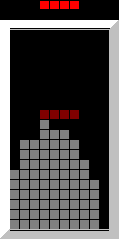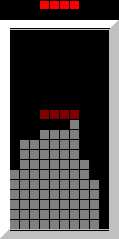The I block can be move if the highest column is anywhere between the 4th and 7th.

In other words, the fifth columns should always be the highest. This allows for the most movement.
In 5 of the 7 blocks the fifth column is ideal.
Here are some techniques to get the fifth column as the highest one.L upwards: If you use irs on the J and L blocks, they will land perfectly, given the stack is flat.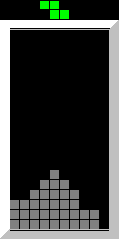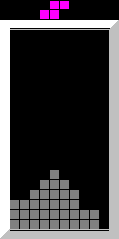Z down the stairs: If you move the S and Z blocks one column to the left or right, they will be placed ideally for 20G.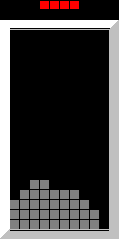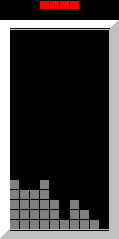5th column I: The I block has to rotated and moved one column to the left. Sometimes you need to do this in reverse order!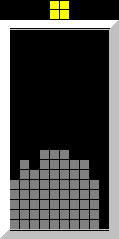The T just has to be rotated twice. The O already lands perfectly and can be moved one space to the left if desired.

 Previous lesson Next lesson Top level